### 二、函数项级数收敛的判别法

1. 收敛与一致收敛

[收敛与收敛区域] un(x)(n=)都是定义在某区间[a,b]上的函数，则称Sn(x)=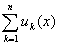n→∞时，都有极限S(x)，即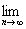Sn(x)==S(x)

rn(x)=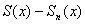rn(x)=0

|rn(x)|<ε或|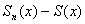|<ε

[一致收敛] 设函数项级数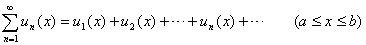|rn(x)|<ε或||<ε